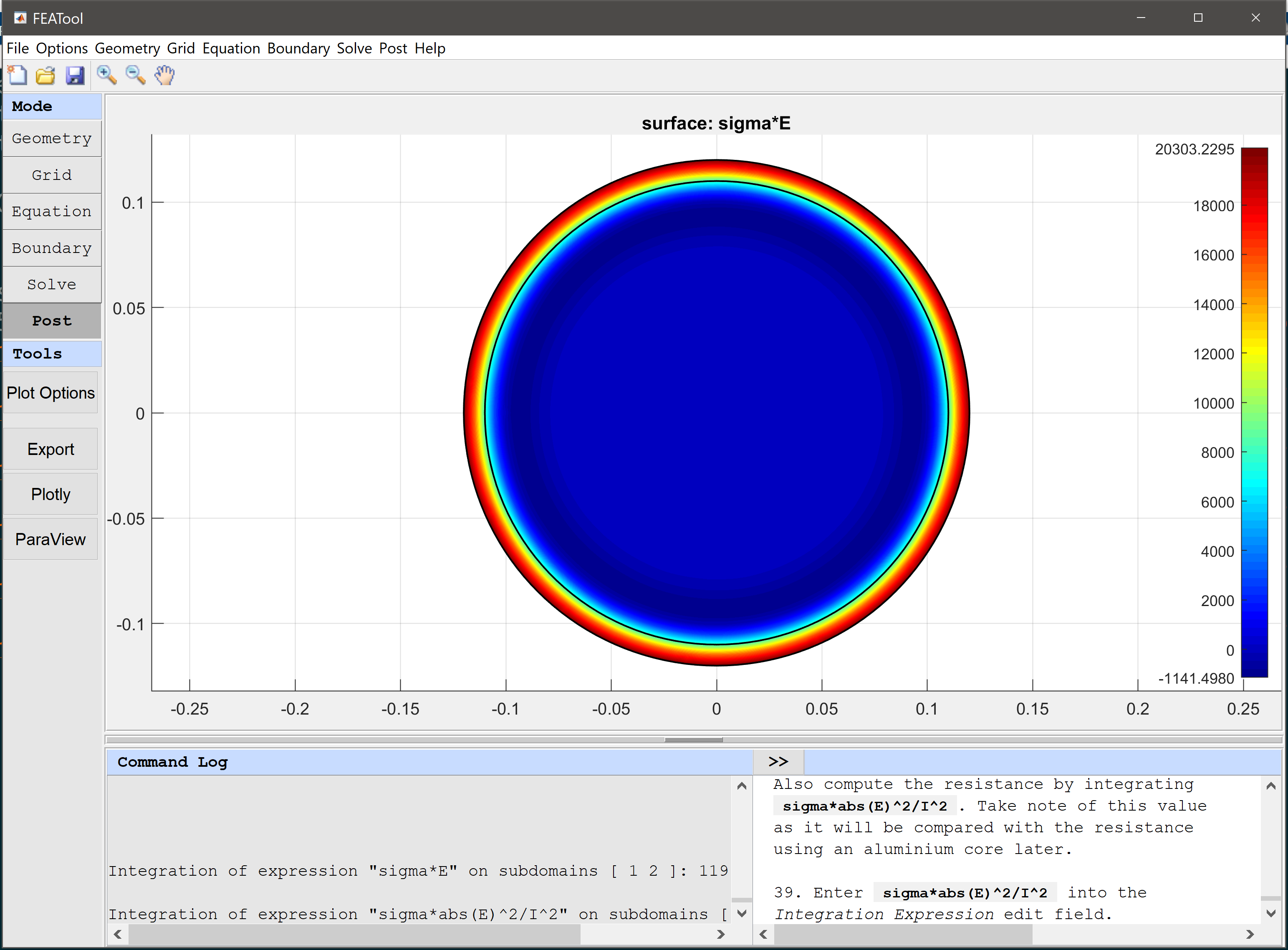# Surface Currents in a Circular WireSkin effect is a phenomenon where an alternating current tends to flow and distribute itself along the surface layer or outside skin of a conductor. This effect can for example be taken advantage of in power transmission applications by layering inexpensive conductor materials (for example aluminium) with a thin layer of more conductive material (like copper). This model examines the skin effect in a two-dimensional cross section of a thick circular wire with an outer radius R = 12 cm.

A time-harmonic complex Helmholtz equation for the amplitude of the electric field E is used to model the skin effect

-∇·(1/µ∇E) + k2E = 0

where mu is the permeability 4π·10-7 H/m, and the wave number k is given by

k = sqrt(iωσ - ω2ε)

where σ is the conductivity of the material, ε the dielectric properties, and ω angular frequency (here 60 Hz). Complex (and imaginary) numbers are denoted using the i coefficient.Tutorial Instructions